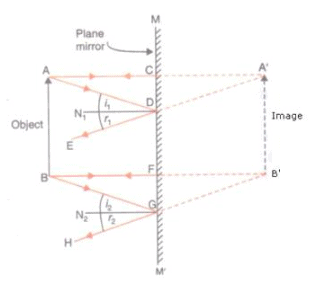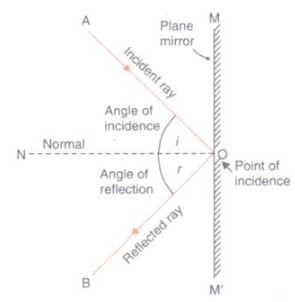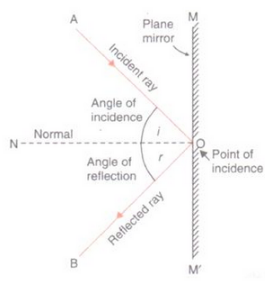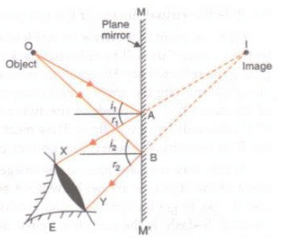# Solutions of Reflection of Light (Page No- 174) - Physics By Lakhmir Singh, Class 10 Class 10 Notes | EduRev

## Class 10 : Solutions of Reflection of Light (Page No- 174) - Physics By Lakhmir Singh, Class 10 Class 10 Notes | EduRev

The document Solutions of Reflection of Light (Page No- 174) - Physics By Lakhmir Singh, Class 10 Class 10 Notes | EduRev is a part of the Class 10 Course Class 10 Physics Solutions By Lakhmir Singh & Manjit Kaur.
All you need of Class 10 at this link: Class 10

Lakhmir Singh Physics Class 10 Solutions Page No:174

Question 21: (a) A wall reflects light and a mirror also reflects light. What difference is there in the way they reflect light ?
(b) Which type of reflection of light leads to the formation of images ?

Solution : (a) A wall has a rough surface, so the reflection by a wall is a diffuse reflection. A parallel beam of light incident on it is reflected in different directions.
A mirror surface is smooth, so the reflection by a mirror is a regular reflection. A parallel beam of light incident on it, gets scattered by making reflected rays in different directions.
(b) Regular reflection

Question 22: What is the difference between regular reflection of light and diffuse reflection of light ? What type of reflection of light takes place from :
(a) a cinema screen (b) a plane mirror (c) a cardboard (d) still water surface of a lake

Solution : In regular reflection, a parallel beam of incident light is reflected as a parallel beam in one direction; while in diffuse reflection, a parallel beam of incident light is reflected in different directions.
(a) Regular reflection
(b) Regular reflection
(c) Diffuse reflection
(d) Regular reflection

Question 23: What can you see in a completely dark room ? If you switch on an electric bulb in this dark room as a light source, explain how you could now see :
(a) the electric bulb.
(b) a piece of white paper.

Solution : When we see in a completely dark room, we are not able to see anything because there is no light in the dark room.
(a) We can see bulb due to the light emitted by the bulb.
(b) We can see a piece of white paper because it reflects the light from the bulb falling on it.

Question 24: (a) A boy with a mouth 5 cm wide stands 2 m away from a plane mirror. Where is his image and how wide is the image of his mouth ?
(b) The boy walks towards the mirror at a speed of 1 m/s. At what speed does his image approach him ?

Solution : (a) The image will form 2 m behind the mirror and the width of the image of boy’s mouth will be 5 cm.
(b) When the boy walks towards the mirror at a speed of 1 m/s, his image will also appear to move towards the mirror at the same speed of 1 m/s. So, the speed at which his image approach him will be 2 m/s + 2 m/s = 4 m/s.

Question 25: (a) An extended object in the form of an arrow pointing upward has been placed in front of a plane mirror. Draw a labelled ray-diagram to show the formation of its image.
(b) State the uses of plane mirrors.

Solution :(b) Uses of Plane mirrors:
(i) Plane mirrors are used to see ourselves. The mirrors on our dressing table and in bathrooms are plane mirrors.
(ii) Plane mirrors are fitted at blind turns of some busy roads so that drivers can see the vehicles coming from the other side and prevent accidents.
(iii) Plane mirrors are used to make periscopes.
(iv) Plane mirrors are fixed on the inside walls of certain shops to make them look bigger.

Question 26: What is meant by ‘reflection of light’ ? Define the following terms used in the study of reflection of light by drawing a labelled ray-diagram :
(a) Incident ray
(b) Point of incidence
(c) Normal
(d) Reflected ray
(e) Angle of incidence
(f) Angle of reflection

Solution : The process of sending back the light rays which fall on the surface of an object is called reflection of light.(a) Incident ray: The ray of light that falls on the mirror surface is called the incident ray.
(b) Point of incidence: The point at which the incident ray falls on the mirror is called the point of incidence.
(c) Normal: The normal is a line at right angle to the mirror surface at the point of incidence.
(d) Reflected ray: The ray of light which is sent back by the mirror is called the reflected rays.
(e) Angle of incidence: The angle of incidence is the angle made by the incident ray with the normal at the point of incidence.
(f) Angle of reflection: The angle of reflection is the angle made by the reflected ray with the normal at the point of incidence.

Question 27: State and explain the laws of reflection of light at a plane surface (like a plane mirror), with the help of a labelled ray-diagram. Mark the angles of ‘incidence’ and ‘reflection’ clearly on the diagram. If the angle of reflection is 47.5°, what will be the angle of incidence ?

Solution : Laws of reflection of light:First law of reflection: According to the first law of reflection, the incidence ray, the reflected ray and the normal (at the point of incidence), all lie in the same plane. For e.g., in the figure, the incident ray AO, the reflected ray OB and the normal ON, all lie in the same plane, the plane of paper.
Second law of reflection: According to the second law of reflection, the angle of reflection is always equal to the angle of incidence. For e.g., if we measure the angle of reflection NOB in the figure, we will find that it is exactly equal to the angle of incidence AON.
If the angle of reflection is 47.5o, the angle of incidence will also be 47.5o in accordance with the second law of reflection.

Question 28: With the help of a labelled ray-diagram, describe how a plane mirror forms an image of a point source of light placed in front of it. State the characteristics of the image formed in a plane mirror.

Solution :Cosider a point source of light O placed in front of a plane mirror MM’. a ray of light OA coming from O in incident at point A on the mirror and gets reflected in the direction AX according to the laws of reflection of light. Another ray of light OB coming from O strikes the mirror ar point B and gets reflected in the direction BY. Rays AX and BY, on producing backwards, meet at point I behind the mirror; which is the image of point source O.
Characteristics of image formed in a plane mirror:
(i) The image formed in a plane mirror is virtual. It cannot be received on a screen.
(ii) The image formed in a plane mirror is erect. It is the same side up as the object.
(iii) The image in a plane mirror is of the same size as the object.
(iv) The image formed by a plane mirror is at the same distance behind the mirror as the object is in front of the mirror.
(v) The image formed by a plane mirror is laterally inverted.

Question 29: (a) Explain why, though both a plane mirror and a sheet of paper reflect light but we can see the image of our face in a plane mirror but not in a sheet of paper.
(b) The image in a plane mirror is virtual and laterally inverted. What does this statement mean ?
(c) Write all the capital letters of the alphabet which look the same in a plane mirror.

Solution : (a) We can see the image of our face in a plane mirror but not in a sheet of paper because images are formed by regular reflection of light and in case of a plane mirror, regular reflection takes place; while in case of a sheet of paper, diffuse reflection takes place.
(b) The image is virtual and laterally inverted means it cannot be obtained on a screen and is reversed sideways.
(c) A, H, I, M, O

Offer running on EduRev: Apply code STAYHOME200 to get INR 200 off on our premium plan EduRev Infinity!

94 docs

,

,

,

,

,

,

,

,

,

,

,

,

,

,

,

,

,

,

,

,

,

,

,

,

;